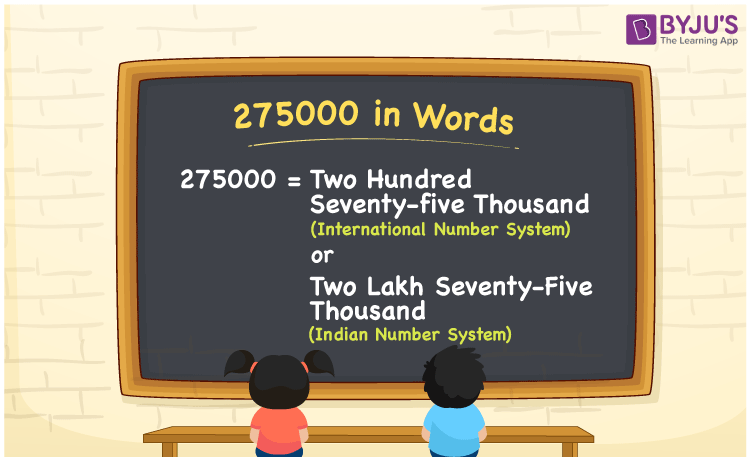# 275000 in Words

275000 in words can be written as Two Hundred Seventy-Five Thousand in the International number system and as Two Lakh Seventy-Five Thousand in the Indian number system. This article will provide complete details of the numbers in words concept which is crucial from the exam perspective. Here, 275000 can be written in both the International and Indian number systems in words.

 275000 in words Two Hundred Seventy-Five Thousand  (or) Two Lakh Seventy-Five Thousand Two Hundred Seventy-Five Thousand  (or) Two Lakh Seventy-Five Thousand in Numbers 275000

## 275000 in English Words## How to Write 275000 in Words?

275000 in words – International number system

The table here indicates the place value chart for 275000 based on the International number system.

 Hundred Thousands Ten Thousands Thousands Hundreds Tens Ones 2 7 5 0 0 0

In expanded form it can be written as

2 x Hundred Thousand  + 7 x Ten Thousand + 5 x Thousand + 0 x Hundred + 0 x Ten + 0 x One

= 2 x 100000 + 7 x 10000 + 5 x 1000 + 0 x 100 + 0 x 10 + 0 x 1

= 200000 + 70000 + 5000

= 275000

= Two Hundred Seventy-Five Thousand

275000 in words – Indian number system

The place value chart of 275000 in the Indian system is given here.

 Lakhs Ten thousands Thousands Hundreds Tens Ones 2 7 5 0 0 0

Here, ones = 0, tens = 0, hundreds = 0, thousands = 5, ten thousands = 7, lakhs = 2

That means, 2 lakhs and 7 ten thousands and 5 thousands

= 2 lakhs + (7 × 10) thousands + 5 thousands

= 2 lakhs + 70 thousands + 5 thousands

= Two Lakh Seventy-Five Thousand

275000 is a natural number that precedes 275001 and succeeds 274999.

275000 in words – Two Hundred Seventy-Five Thousand (or) Two Lakh Seventy-Five Thousand

Is 275000 an odd number? – No

Is 275000 an even number? – Yes

Is 275000 a perfect square number? – No

Is 275000 a perfect cube number? – No

Is 275000 a prime number? – No

Is 275000 a composite number? – Yes

## Frequently Asked Questions on 275000 in Words

### How do you write 275000 in words?

275000 can be written in words as “Two Hundred Seventy-Five Thousand (or) Two Lakh Seventy-Five Thousand”.

### Write Two Hundred Seventy-Five Thousand (or) Two Lakh Seventy-Five Thousand in numbers.

Two Hundred Seventy-Five Thousand (or) Two Lakh Seventy-Five Thousand can be written in numbers as 275000.

### Is 275000 an odd number?

No, 275000 is not an odd number as it is completely divisible by 2.